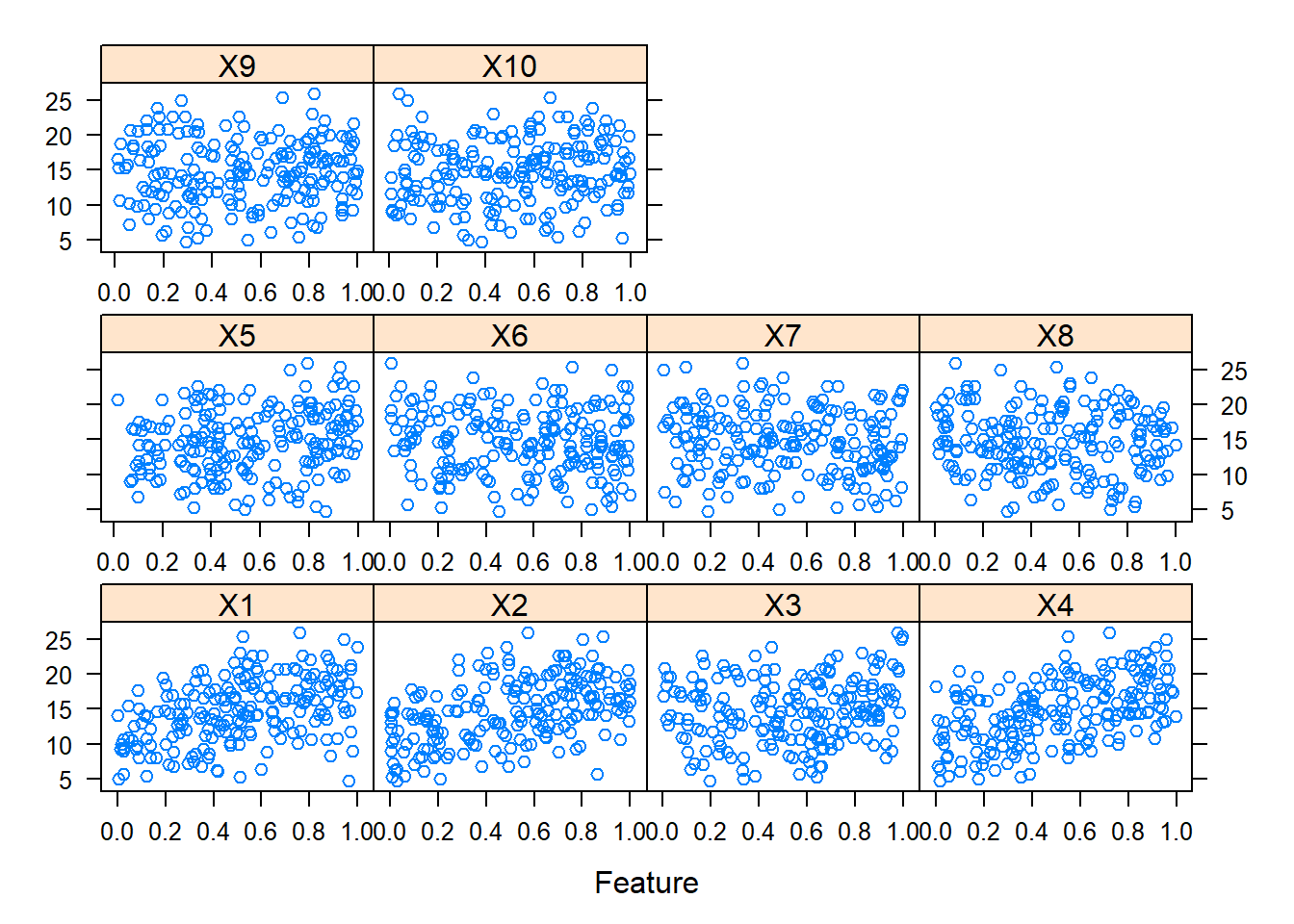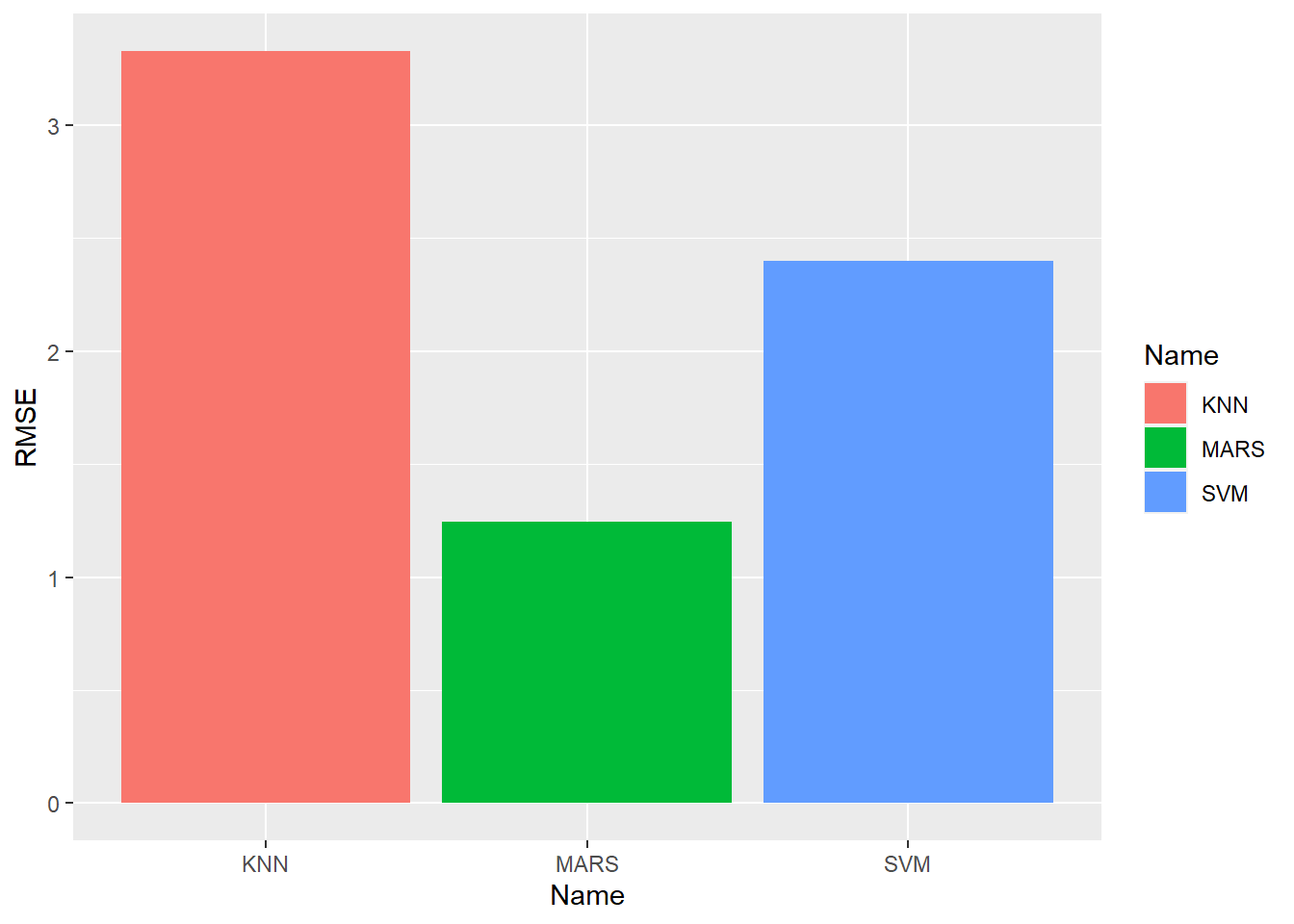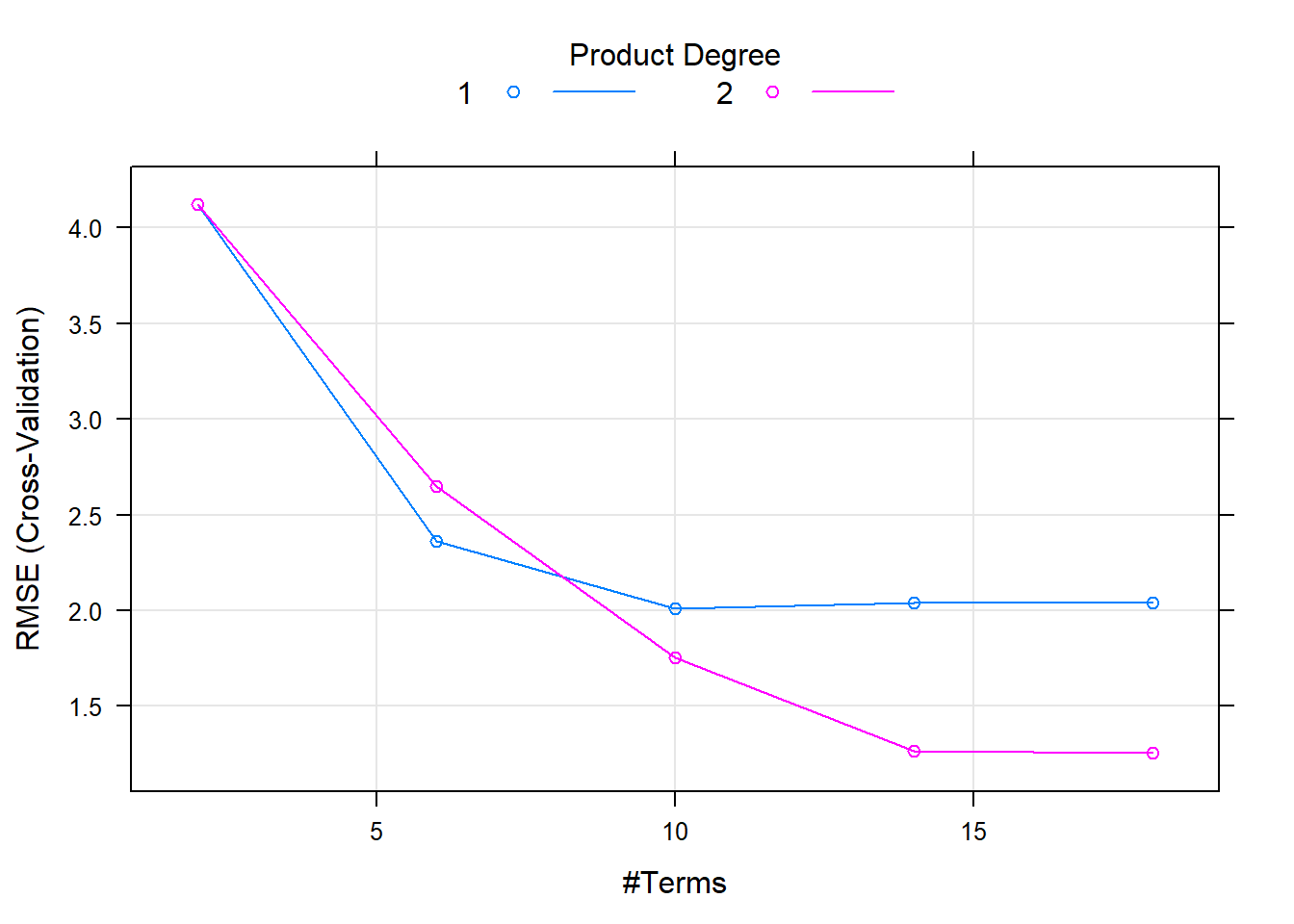title: “HMWK8 7.2 HH” author: “Hui (Gracie) Han” output: html_document —

getwd()
##  "T:/00-624 HH Predictive Analytics/HMWK8 NonLinearRegresn/HMWK8 HH"
# clear the workspace
rm(list = ls())
library(mlbench)
library(caret)
## Loading required package: lattice
## Loading required package: ggplot2
library(earth)
## Loading required package: Formula
## Loading required package: plotmo
## Loading required package: plotrix
## Loading required package: TeachingDemos
library(kernlab)
##
## Attaching package: 'kernlab'
## The following object is masked from 'package:ggplot2':
##
##     alpha
library(nnet)
library(ggplot2)
library(mice)
##
## Attaching package: 'mice'
## The following objects are masked from 'package:base':
##
##     cbind, rbind
library(tidyverse)
## -- Attaching packages --------------------------------------- tidyverse 1.3.0 --
## v tibble  3.0.4     v dplyr   1.0.2
## v tidyr   1.1.2     v stringr 1.4.0
## v readr   1.4.0     v forcats 0.5.0
## v purrr   0.3.4
## -- Conflicts ------------------------------------------ tidyverse_conflicts() --
## x purrr::lift()    masks caret::lift()
library(kableExtra)
##
## Attaching package: 'kableExtra'
## The following object is masked from 'package:dplyr':
##
##     group_rows

## 7.2

Exercises 7.2 & 7.5 from the K&J book. Friedman (1991) introduced several benchmark data sets create by simulation. One of these simulations used the following nonlinear equation to create data:

y=10sin(πx1x2)+20(x3−0.5)2+10x4+5x5+N(0,σ2)

where the x values are random variables uniformly distributed between [0, 1] (there are also 5 other non-informative variables also created in the simulation). The package mlbench contains a function called mlbench.friedman1 that simulates these data:

set.seed(160)
trainingData = mlbench.friedman1(200, sd=1)
trainingData$x = data.frame(trainingData$x)
testData = mlbench.friedman1(5000, sd=1)
testData$x = data.frame(testData$x)
featurePlot(trainingData$x, trainingData$y)### Function for Future Models

We are creating a fuctnion model.eval that will be applied to the future model tuning on this data.

model.eval = function(modelmethod, gridSearch = NULL)
{
Model = train(x = trainingData$x, y = trainingData$y,
method = modelmethod,
tuneGrid = gridSearch,
preProcess = c('center', 'scale'),
trControl = trainControl(method='cv'))

Pred = predict(Model, newdata = testData$x) modelperf = postResample(Pred, testData$y)
print(modelperf)
}

### K-Nearest Neighbors

perfknn = model.eval('knn')
##      RMSE  Rsquared       MAE
## 3.3269737 0.6019938 2.6578992

### Support Vector Machine

perfsvm = model.eval('svmRadial')
##      RMSE  Rsquared       MAE
## 2.3974830 0.7921619 1.8581947

marsGrid = expand.grid(degree = 1:2, nprune = seq(2, 20, by=4))
perfmars = model.eval('earth', marsGrid)
##      RMSE  Rsquared       MAE
## 1.2470958 0.9372554 0.9916533

After applying the function to several models, the RMSE from these models are displayed side by side.

df.perf = rbind(data.frame(Name = 'KNN', RMSE = perfknn),
data.frame(Name = 'SVM', RMSE = perfsvm),
data.frame(Name = 'MARS', RMSE = perfmars))

ggplot() +
geom_bar(data = df.perf, aes(x = Name, y = RMSE, fill=Name), stat="identity")### Optimum Model

Question: Which models appear to give the best performance? The MARS model give us the lowest RMSE,lower than the KNN and SVM models. So, MARS is our optimum model, among three.

### X1-X5 in MARS

Question: Does MARS select informative predictors (those named X1-X5)

set.seed(201)
marsGrid = expand.grid(degree = 1:2, nprune = seq(2, 20, by=4))

MARSModel = train(x = trainingData$x, y = trainingData$y,
method = 'earth',
# method='bagEarth',
tuneGrid = marsGrid,
preProcess = c('center', 'scale'),
trControl = trainControl(method='cv'))
MARSModel
## Multivariate Adaptive Regression Spline
##
## 200 samples
##  10 predictor
##
## Pre-processing: centered (10), scaled (10)
## Resampling: Cross-Validated (10 fold)
## Summary of sample sizes: 180, 180, 180, 180, 180, 180, ...
## Resampling results across tuning parameters:
##
##   degree  nprune  RMSE      Rsquared   MAE
##   1        2      4.119515  0.2080549  3.4285363
##   1        6      2.364352  0.7383600  1.8901997
##   1       10      2.009247  0.8114766  1.6084995
##   1       14      2.042420  0.8059495  1.6342658
##   1       18      2.042420  0.8059495  1.6342658
##   2        2      4.119515  0.2080549  3.4285363
##   2        6      2.647151  0.6693283  2.1291033
##   2       10      1.754305  0.8614559  1.4043137
##   2       14      1.266577  0.9246299  1.0055150
##   2       18      1.254487  0.9276409  0.9885292
##
## RMSE was used to select the optimal model using the smallest value.
## The final values used for the model were nprune = 18 and degree = 2.
varImp(MARSModel)
## earth variable importance
##
##    Overall
## X4  100.00
## X1   74.56
## X2   45.99
## X3   12.91
## X5    0.00

Yes, our model as MARS correctly identies that the X1, X2, X3, X4 are important variables. X4 is the most important among all of them. The rest of the variables (X6, X7, X9, X9, X10) are not important in our MARS model.

plot(MARSModel)At both the 1st product degree and 2nd product degree models, the model performance improves as numbers of terms increase, at the degree and patten similar to both. When there are 1 terms, the RMSE starts at 4.1, and then gradually decreases as numbers of terms are increased. aT THE 6 terms, the RMSE drops to 2.0 in both 1st and 2nd degree models. Further increasing of the numbers of terms thereafter do not seem to improve the model performance too much.